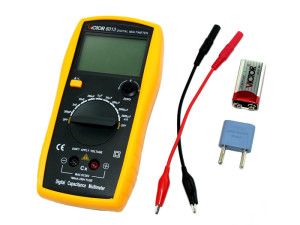# Capacitance Meter Fluke

### Digital Capacitance Meter Tester – Capacitor Testercapacitance meter is a piece of electronic test equipment used to measure capacitance, mainly of discrete capacitors. Depending on the sophistication of the meter, it may display the capacitance only, or it may also measure a number of other parameters such as leakage, equivalent series resistance (ESR), and inductance. For most purposes and in most cases the capacitor must be disconnected from circuit; ESR can usually be measured in circuit.

## Shop For Capacitance Meters

Some checks can be made without a specialized instrument, particularly on aluminium electrolytic capacitors which tend to be of high capacitance and to be subject to poor leakage. A multimeter in a resistance range can detect a short-circuited capacitor (very low resistance) or one with very high leakage (high resistance, but lower than it should be; an ideal capacitor has infinite DC resistance). A crude idea of the capacitance can be derived with an analog multimeter in a high resistance range by observing the needle when first connected; current will flow to charge the capacitor and the needle will “kick” from infinite indicated resistance to a relatively low value, and then drift up to infinity. The amplitude of the kick is an indication of capacitance. Interpreting results requires some experience, or comparison with a good capacitor, and depends upon the particular meter and range used.

Capacitance is the ability of a body to store an electrical charge. Any object that can be electrically charged exhibits capacitance. A common form of energy storage device is a parallel-plate capacitor. In a parallel plate capacitor, capacitance is directly proportional to the surface area of the conductor plates and inversely proportional to the separation distance between the plates. If the charges on the plates are +q and −q, and V gives the voltage between the plates, then the capacitance C is given by

Many DVMs (digital volt meters) have a capacitance-measuring function. These usually operate by charging and discharging the capacitor under test with a known current and measuring the rate of rise of the resulting voltage; the slower the rate of rise, the larger the capacitance. DVMs can usually measure capacitance from nanofarads to a few hundred microfarads, but wider ranges are not unusual.

It is also possible to measure capacitance by passing a known high-frequency alternating current through the device under test and measuring the resulting voltage across it (does not work for polarised capacitors).

When troubleshooting circuit problems, a few problems are intermittent or only show up with the working voltage applied, and are not revealed by measurements with equipment, however sophisticated, which uses low test voltages. Some problems are revealed by using a “freezer” spray and observing the effect on circuit operation. Ultimately, in difficult cases routine replacement of capacitors (relatively cheap components) is easier than arranging measurements of all relevant parameters in working conditions.

Some more specialised instruments measure capacitance over a wide range using the techniques described above, and can also measure other parameters. Low stray and parasitic capacitance can be measured if a low enough range is available. Leakage current is measured by applying a direct voltage and measuring the current in the normal way.